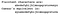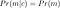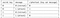# An introduction to secure communication

In this post we will see the most basic example for encrypting your messages, then we will show that this by no means is secure and finally we will introduce what it means to have a perfect secrecy scheme and why is not practical. Then, we’ll get to see what are stream ciphers for symmetric encryption. Python code for this post can be found here.

One of the main objectives of cryptography is to enable secure communication between a sender and a receiver. This means that if someone is intercepting (eavesdropping) the ciphertexts (encrypted messages) sent between parties A and B he is not able to get any information. To introduce nomenclature I will show the most basic cipher, the shift cipher.

# Shift cipher

In the shift cipher (a.k.a Caesar’s cipher), two parties A and B agree on a common key, a number in between 0 to 24, this key is secret and they don’t share it with anybody else. In the shift cipher the key is translated into the number of jumps on the alphabet, let’s take a key (shift) of 3 as an example. The substitution of letters would be:Caesar’s cipher substitution for key k=3

Now imagine that A wants to send to B the message “let the people vote” (a.k.a the message in plaintext), but obviously A cannot send this message as is through an insecure communication channel so he has to transform the message to the encrypted space as “ohw wkh shrsoh yrwh” (this is known as the ciphertext), that is, it substitutes “l” by “o”, “e” by “h” and so on as depicted in the substitution image above for key=3. Then he can send the ciphertext through the insecure channel and people eavesdropping this are not able to decrypt (reveal) the original message unless they have the secret key.

This cipher is very simple, to crack it the eavesdropper just needs to try all the possible secret keys (shifts) from 0 to 24. For instance if an eavesdropper C tried to decrypt the message using the key=1 he would have the decrypted message “ngv vjg rgqrng xqvg” when observing the previous ciphertext (you can try it easily in this webpage), obviously this message does not make any sense in english so he’d think that this is not the correct key and would try a new one. It is easy to see that it won’t take him very long to find that the original key for encryption was 3 and therefore decrypt all the messages that he was able to grasp between A and B.

# Better security by increasing the key space

We’ve seen that if the key space (number of possible secret keys) is small it is not difficult to find the correct key and then decrypt all the messages. So, we can increase this key size and then the probability for the attacker to find the correct key is reduced (or, he’ll have to invest a lot of time to find it).

In the practical example I will show in this section (have a look at the code in the notebook) we are going to work with an improved cipher, called the mono-alphabetic substitution cipher. In this cipher we substitute the letters “a, b, c,..., z” with a random permutation of those. For instance a key can be the one depicted on the image below:

in this case the letters “a”, “b”, “c” … from the message are mapped to the corresponding values below “a”, “v”, “s”… It is a simple substitution. The key space of this cipher is much larger compared to the Shift cipher, we can in fact generate 24!=24*23*22…*2*1=620448401733239439360000 possible keys. Looks pretty secure, right?. Brute force trial and error of the keys would take long (the probability of guessing the correct key in 1 trial is (1/24!=1.61E-24) and it doesn’t seem practical.

# Leaking information from ciphertexts

In the mono-alphabetic cipher it may be difficult for the attacker to get the exact key but still he can get some valuable information by just looking at the ciphertexts. Imagine that the attacker knows the language of communication in between the two parties (Alice and Bob) and so by just observing the ciphertexts he can infer information from the messages. Imagine this language is plain english, the attacker then knows that some words are more frequent than others, for instance “the”, “be”, “to”, “of” or “and” are listed as the most frequent words in english. This means that the attacker will observe many times the words “dco”, “vo”, “dk”, “ki” and “agb” in the ciphertexts (if we use the substitution cipher introduced in the example). Now he can exploit that to find some letter substitutions in the key.

In the example of the notebook I used a much simpler but similar attack. In this, I used text of the famous George Orwell’s book 1984. First I calculated the frequencies of letters (not words as before) taking all the words in the book and got ‘a’ appearing 36548, ‘b’ 7668 times, ‘c’: 11642 times, ‘d’: 19033… in sorted order. This is my source of truth for frequency of letters in the english language. Then, in order to estimate a regular message in plain english I sampled a chunk of 5% length of the book. With this chunk we calculate the ciphertext (the simple substitution above) and compute the frequencies of the words on it. Now, just by comparing the frequencies in the ciphertext and those of the english language we have been able to estimate 8 correct substitutions of the letters out of 24. How? Just by having a closer look at the ciphertexts with the prior that we know the language of communication is english. The conclusion: One can infer information from the original message just by looking at the ciphertext. This is an unwanted result.

# Perfect secrecy and the one time pad

Can we find a way such that the ciphertext does not contain any information?. First let me write a definition (a more formal definition can be found in the book of Katz and Lindell) . An encryption scheme is considered to be perfectly secret ifAn encryption scheme is perfectly secret if the probability of a message does not change by the observation of a ciphertext.

where m represents all possible messages and c all possible ciphertexts. This means that the probability of finding a specific message does not change by the observation of any cipertext, i.e. the ciphertext does not contain any information about the message.

There’s a way to achieve perfect secrecy, and this is through one time pad. Let me explain it with a simple example. Imagine the scenario where Bob is a submarine captain of a secret army and Alice is his contact on the mainland. In the next mission Bob is told to go to the enemy base and wait for a communication from Alice of “attack” or “retreat” at exactly 4 p.m. They therefore want to communicate with messages of 1 bit, (1 means attack and 0 means retreat). The enemy has been informed by one of his spies that Bob is going to his base at 4 p.m and will be waiting for orders from Alice. He is also aware of the code they use. If the enemy knows that Bob is not attacking he will let him go (let’s assume the enemy is much weaker than Bob), otherwise he will try to attack first with all his force.

The first thing that Alice and Bob do is to meet in person at the base and agree on a common key for communication, this key has to be the same length as the message they want to send (in our case one bit). Then they agree that they calculate the ciphertext by XORing the message with the key and do again XOR for decrypting the message (recall that XOR is the same as to apply addition modulo 2 operation). In the following table it is represented the encryption of one bit using XORXOR operation for the one time pad for messages of 1 bit.

Once they agreed on a key (and are 100% sure nobody else knows it) they can communicate once through an insecure channel. Le’t say the key is 1. If Alice wants Bob to attack, she will send the ciphertext 0, otherwise 1. Now the attacker can observe this ciphertext but has no information whatsoever on the key, let’s say he observes ciphertext=1, from the above he can say that if the secret key is 0 then the order is attack but if it is 1 the order is retreat so P(attack)=P(retreat)=0.5. So he gets exactly the same probability for either attack or retreat, that means the ciphertext does not contain any information and he hasn’t learned anything new from the intentions of the secret army. Further explanation and implementation in Python can be found here.

The one time pad can be extended to many bits, for instance if the message is of 256 bits, the binary key has to be of 256 bits too because we are masking 1 bit by 1 bit (this is a requirement from Shannon’s theorem for perfect secrecy found on this book). It is important to notice however that the key can only be used to transmit one message. If more messages were transmitted with the same key one could start computing the frequencies of the bits and eventually make statistics similar to what we did in the previous section.

One time pads are not practical for implementation because of the following reasons:

• The key has to be at least as long as the message one wants to transmit. This means we have to store a lot of information. For instance if we transmit a text message in ASCII encoding (8 bits per letter) and send a word of 10 letters we would need a key of 80 bits at least.
• For perfect secrecy one has to use a new key every time. When Alice and Bob meet in person they will have to exchange a lot of keys and use one by one sequentially for their communications. This again is a lot of information and is impractical.
• Alice and Bob have to make sure that they are the only ones that know the key. In the examples I always stated that they have to meet in person, this is a way to make sure that nobody is spying them. When using computers one wants to establish a secure communication through an insecure channel like the internet between two computers that are physically very far away. This makes the one time pad totally impractical.

# Improving the one time pad: stream ciphers

The general idea of the one time pad is used in stream ciphers. Here we need the notion of a pseudorandom generator (see Introduction to modern cryptography from Katz and Lindell), this is an algorithm that inputs a number (a.k.a the seed) and outputs what looks like a random string of bits. In essence a good pseudorandom generator (PRG) must output bit strings that are difficult to distinguish from pure random.

The PRG algorithm and the seed (the secret key) are shared among Alice and Bob so they can generate the same stream of “close-to-random” bits. These stream is used to pad the message and encrypt/decrypt the same way we did (XORing) in the one time pad in the previous section. Yes!, this is very similar to the one time pad!. But not exactly the same…

As expected there’s no free lunch. PRGs do not produce pure randomness. Pure randomness would mean that if one observes the output of n bits produced by the PRG (without knowing the seed) the probability of observing either 1 or 0 on the next bit generated by the PRG is exactly 0.5. This can’t be the case because the PRGs are deterministic algorithms. So there’s still information leakage from the ciphertext.

Alice and Bob can generate exactly the same stream of bits if they know both, the PRG algorithm and the seed to initiate it. To an adversary (knowing the PRG algorithm but not the seed) it is very difficult do guess the sequence computationally speaking so we would say this is secure. We can even measure the security of the stream cipher by comparing different PRGs, i.e. one stream cipher is more secure than another stream cipher if the pseudo-random numbers generated by the first looks more random (informally speaking) to an observer looking at the generated bits of both.

And finally good news! stream ciphers are used in modern day communications!. For instance A5 is used in cell phone communications but as we said it is not perfectly secure since the PRG generates pseudo-random deterministic bits that are not entirely random.

I won’t extend on stream ciphers here but I hope you got the general idea. For further details have a look at the lecture of Prof. Christof Paar on the topic. He approaches the topic differently, first explaining stream ciphers and later on perfect secrecy. Another excellent reference is the book of Katz and Lindell.

# Improving the stream cipher using quantum physics

Now imagine that Alice and Bob could generate the same pure random streams of bits for a moment. For an attacker eavesdropping all the communications between Alice and Bob the ciphertexts would look totally random, that’s the case of perfect secrecy!. Don’t overreact but we are close to find the perfect cipher!. Now the question. Can we generate pure correlated randomness between Alice and Bob?

Let’s think how we can generate pure randomness first. Just use a physical process like thermal fluctuation on a CPU of your computer. Say for instance that normally the CPU is at X temperature, then if at the moment of generating one random bit we measure the temperature and is below X we output 0 otherwise 1. A better way to generate random noise is to prepare a quantum state for an electron in which the probability when measuring its spin is exactly 0.5 for up or down.

Ok, we got ways using physics to generate pure randomness on Alice and Bob’s ends. However you have to remember that Alice and Bob have to generate exactly the same stream of bits i.e. the same randomness. We cannot achieve this using classical thermal fluctuation so Alice and Bob need to have some sort of correlation between them. Using the properties of quantum entanglement (see quantum key distribution) Alice and Bob and can prepare two physical quantum states on their end that are “correlated” so they can both generate the same pure randomness (again this comes from the random nature of quantum physics).

Yes… this is far beyond what I wanted to explain in this post, but I come from a physics background and it was very tempting to at least mention.

# Takeaways from this post

We presented the shift cipher and the substitution cipher as simple examples to illustrate the problem of the ciphertext carrying information from the message. We’ve seen with a simple attack on those ciphers how can one get information from the messages by just observing the ciphertexts. Then stated the problem of getting ciphertexts not containing any information from the message, i.e. perfect secrecy and we’ve seen that even though we can achieve it with the one time pad this is not a good practical solution for several reasons. A more practical approach is to use stream ciphers where take the same philosophy of padding the message with a random stream of bits. Here however we use pseudo-random generators to generate the noise, and the problem is that this noise is not purely random so stream ciphers are not perfectly secure but practical in many applications such as mobile communications. One way to make stream ciphers perfectly secure is to generate the randomness using quantum physics, an active field of research nowadays.

Please if you liked, give me some claps on medium or star my repository on Github.

PhD in Physics, AI Research Engineer

## More from Sebastia Agramunt-Puig

PhD in Physics, AI Research Engineer